# Search by Topic

#### Resources tagged with Visualising similar to Reflecting Squarely:

Filter by: Content type:
Age range:
Challenge level:

### There are 176 results

Broad Topics > Using, Applying and Reasoning about Mathematics > Visualising### Reflecting Squarely

##### Age 11 to 14 Challenge Level:

In how many ways can you fit all three pieces together to make shapes with line symmetry?##### Age 11 to 14 Challenge Level:

How many different symmetrical shapes can you make by shading triangles or squares?### Troublesome Dice

##### Age 11 to 14 Challenge Level:

When dice land edge-up, we usually roll again. But what if we didn't...?### Pattern Power

##### Age 5 to 14

Mathematics is the study of patterns. Studying pattern is an opportunity to observe, hypothesise, experiment, discover and create.### On the Edge

##### Age 11 to 14 Challenge Level:

If you move the tiles around, can you make squares with different coloured edges?### Squares in Rectangles

##### Age 11 to 14 Challenge Level:

A 2 by 3 rectangle contains 8 squares and a 3 by 4 rectangle contains 20 squares. What size rectangle(s) contain(s) exactly 100 squares? Can you find them all?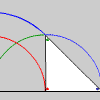### Turning Triangles

##### Age 11 to 14 Challenge Level:

A triangle ABC resting on a horizontal line is "rolled" along the line. Describe the paths of each of the vertices and the relationships between them and the original triangle.### Triangles to Tetrahedra

##### Age 11 to 14 Challenge Level:

Imagine you have an unlimited number of four types of triangle. How many different tetrahedra can you make?### Frogs

##### Age 11 to 14 Challenge Level:

How many moves does it take to swap over some red and blue frogs? Do you have a method?### Isosceles Triangles

##### Age 11 to 14 Challenge Level:

Draw some isosceles triangles with an area of $9$cm$^2$ and a vertex at (20,20). If all the vertices must have whole number coordinates, how many is it possible to draw?### Weighty Problem

##### Age 11 to 14 Challenge Level:

The diagram shows a very heavy kitchen cabinet. It cannot be lifted but it can be pivoted around a corner. The task is to move it, without sliding, in a series of turns about the corners so that it. . . .##### Age 11 to 14 Challenge Level:

Four rods, two of length a and two of length b, are linked to form a kite. The linkage is moveable so that the angles change. What is the maximum area of the kite?### Framed

##### Age 11 to 14 Challenge Level:

Seven small rectangular pictures have one inch wide frames. The frames are removed and the pictures are fitted together like a jigsaw to make a rectangle of length 12 inches. Find the dimensions of. . . .### Tetra Square

##### Age 11 to 14 Challenge Level:

ABCD is a regular tetrahedron and the points P, Q, R and S are the midpoints of the edges AB, BD, CD and CA. Prove that PQRS is a square.### Take Ten

##### Age 11 to 14 Challenge Level:

Is it possible to remove ten unit cubes from a 3 by 3 by 3 cube so that the surface area of the remaining solid is the same as the surface area of the original?### Clocked

##### Age 11 to 14 Challenge Level:

Is it possible to rearrange the numbers 1,2......12 around a clock face in such a way that every two numbers in adjacent positions differ by any of 3, 4 or 5 hours?### Trice

##### Age 11 to 14 Challenge Level:

ABCDEFGH is a 3 by 3 by 3 cube. Point P is 1/3 along AB (that is AP : PB = 1 : 2), point Q is 1/3 along GH and point R is 1/3 along ED. What is the area of the triangle PQR?### Icosian Game

##### Age 11 to 14 Challenge Level:

This problem is about investigating whether it is possible to start at one vertex of a platonic solid and visit every other vertex once only returning to the vertex you started at.### Hidden Rectangles

##### Age 11 to 14 Challenge Level:

Rectangles are considered different if they vary in size or have different locations. How many different rectangles can be drawn on a chessboard?### An Unusual Shape

##### Age 11 to 14 Challenge Level:

Can you maximise the area available to a grazing goat?### Cutting a Cube

##### Age 11 to 14 Challenge Level:

A half-cube is cut into two pieces by a plane through the long diagonal and at right angles to it. Can you draw a net of these pieces? Are they identical?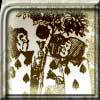### Painting Cubes

##### Age 11 to 14 Challenge Level:

Imagine you have six different colours of paint. You paint a cube using a different colour for each of the six faces. How many different cubes can be painted using the same set of six colours?### Convex Polygons

##### Age 11 to 14 Challenge Level:

Show that among the interior angles of a convex polygon there cannot be more than three acute angles.### Cubes Within Cubes

##### Age 7 to 14 Challenge Level:

We start with one yellow cube and build around it to make a 3x3x3 cube with red cubes. Then we build around that red cube with blue cubes and so on. How many cubes of each colour have we used?### Coloured Edges

##### Age 11 to 14 Challenge Level:

The whole set of tiles is used to make a square. This has a green and blue border. There are no green or blue tiles anywhere in the square except on this border. How many tiles are there in the set?### Zooming in on the Squares

##### Age 7 to 14

Start with a large square, join the midpoints of its sides, you'll see four right angled triangles. Remove these triangles, a second square is left. Repeat the operation. What happens?### Sea Defences

##### Age 7 to 14 Challenge Level:

These are pictures of the sea defences at New Brighton. Can you work out what a basic shape might be in both images of the sea wall and work out a way they might fit together?### Paving Paths

##### Age 11 to 14 Challenge Level:

How many different ways can I lay 10 paving slabs, each 2 foot by 1 foot, to make a path 2 foot wide and 10 foot long from my back door into my garden, without cutting any of the paving slabs?### All in the Mind

##### Age 11 to 14 Challenge Level:

Imagine you are suspending a cube from one vertex and allowing it to hang freely. What shape does the surface of the water make around the cube?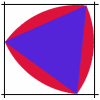### Rolling Triangle

##### Age 11 to 14 Challenge Level:

The triangle ABC is equilateral. The arc AB has centre C, the arc BC has centre A and the arc CA has centre B. Explain how and why this shape can roll along between two parallel tracks.### Rati-o

##### Age 11 to 14 Challenge Level:

Points P, Q, R and S each divide the sides AB, BC, CD and DA respectively in the ratio of 2 : 1. Join the points. What is the area of the parallelogram PQRS in relation to the original rectangle?### Picturing Triangular Numbers

##### Age 11 to 14 Challenge Level:

Triangular numbers can be represented by a triangular array of squares. What do you notice about the sum of identical triangle numbers?### Cubes Within Cubes Revisited

##### Age 11 to 14 Challenge Level:

Imagine starting with one yellow cube and covering it all over with a single layer of red cubes, and then covering that cube with a layer of blue cubes. How many red and blue cubes would you need?### Seven Squares

##### Age 11 to 14 Challenge Level:

Watch these videos to see how Phoebe, Alice and Luke chose to draw 7 squares. How would they draw 100?### Route to Infinity

##### Age 11 to 14 Challenge Level:

Can you describe this route to infinity? Where will the arrows take you next?### 3D Stacks

##### Age 7 to 14 Challenge Level:

Can you find a way of representing these arrangements of balls?### Auditorium Steps

##### Age 7 to 14 Challenge Level:

What is the shape of wrapping paper that you would need to completely wrap this model?### Polygon Rings

##### Age 11 to 14 Challenge Level:

Join pentagons together edge to edge. Will they form a ring?### Diminishing Returns

##### Age 11 to 14 Challenge Level:

How much of the square is coloured blue? How will the pattern continue?### Christmas Chocolates

##### Age 11 to 14 Challenge Level:

How could Penny, Tom and Matthew work out how many chocolates there are in different sized boxes?### Eight Hidden Squares

##### Age 7 to 14 Challenge Level:

On the graph there are 28 marked points. These points all mark the vertices (corners) of eight hidden squares. Can you find the eight hidden squares?### There and Back Again

##### Age 11 to 14 Challenge Level:

Bilbo goes on an adventure, before arriving back home. Using the information given about his journey, can you work out where Bilbo lives?### Keep Your Distance

##### Age 11 to 14 Challenge Level:

Can you mark 4 points on a flat surface so that there are only two different distances between them?### Square Coordinates

##### Age 11 to 14 Challenge Level:

A tilted square is a square with no horizontal sides. Can you devise a general instruction for the construction of a square when you are given just one of its sides?### Picturing Square Numbers

##### Age 11 to 14 Challenge Level:

Square numbers can be represented as the sum of consecutive odd numbers. What is the sum of 1 + 3 + ..... + 149 + 151 + 153?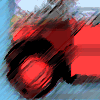### Buses

##### Age 11 to 14 Challenge Level:

A bus route has a total duration of 40 minutes. Every 10 minutes, two buses set out, one from each end. How many buses will one bus meet on its way from one end to the other end?### Cuboids

##### Age 11 to 14 Challenge Level:

Find a cuboid (with edges of integer values) that has a surface area of exactly 100 square units. Is there more than one? Can you find them all?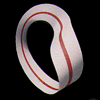### Bands and Bridges: Bringing Topology Back

##### Age 7 to 14

Lyndon Baker describes how the Mobius strip and Euler's law can introduce pupils to the idea of topology.### Coordinate Patterns

##### Age 11 to 14 Challenge Level:

Charlie and Alison have been drawing patterns on coordinate grids. Can you picture where the patterns lead?### Square It

##### Age 11 to 16 Challenge Level:

Players take it in turns to choose a dot on the grid. The winner is the first to have four dots that can be joined to form a square.